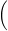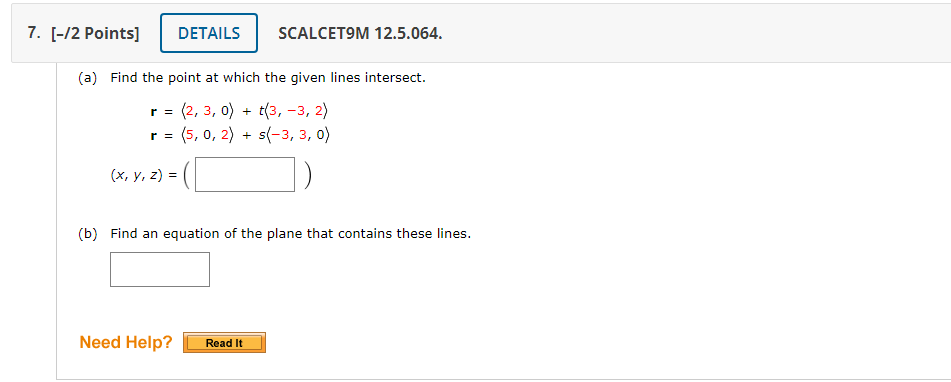# Get help with vectors and spacesRecent questions in Vectors and spaces
Vectors and spaces

###(a) Find the point at which the given lines intersect. r  =2, 3, 0+  t3, −3, 2r  =5, 0, 2+  s−3, 3, 0(x, y, z) =(b) Find an equation of the plane that contains these lines.Vectors and spaces
ANSWERED### Use the figure shawn 10 identify each a. vertical sngles b. adjacent angles c. linear pairs d. supplementary angles e. complementary angles

Vectors and spaces
ANSWERED### Solve the system of equations $$\begin{cases}2x+3y=5\\5x−4y=2\end{cases}$$

Vectors and spaces
ANSWERED### Determine whether or not F is a conservative vector field. If it is, find a function f such that F=delf. $$\displaystyle{F}{\left({x},{y}\right)}={\left({3}{x}^{{2}}-{2}{y}^{{2}}\right)}{i}+{\left({4}{x}{y}+{3}\right)}{j}$$

Vectors and spaces
ANSWERED### (a) Find a nonzero vector orthogonal l to the plane the points P, Q, and R, and (b) find the area of triangle PQR $$P(1,0,1),Q(-2,1,3), R(4,2,5)$$

Vectors and spaces
ANSWERED### The set $$B=\left\{1+t^{2}, t+t^{2}, 1+2t+t^{2}\right\}$$ is a basis for $$\displaystyle{\mathbb{{{P}}}}_{{{2}}}$$. Find the coordinate vector of $$\displaystyle{p}{\left({t}\right)}={1}+{4}{t}+{7}{t}^{{{2}}}$$ relative to B.

Vectors and spaces
ANSWERED### Find the domain of the vector function. $$\displaystyle{r}{\left({t}\right)}={t}-\frac{{2}}{{t}}+{2}{i}+{\sin{{t}}}{j}+{\ln{{\left({9}-{t}^{{2}}\right)}}}{k}$$

Vectors and spaces
ANSWERED### Find the volume of the parallelepiped with adjacent edges PQ, PR, and PS. $$P(3, 0, 1), Q(-1, 2, 5), R(5, 1, -1), S(0, 4, 2)$$

Vectors and spaces
ANSWERED### Use the cross product to find the sine of the angle between the vectors $$\displaystyle{u}={\left({2},{3},-{6}\right)}$$ and $$v =(2,3,6)$$.

Vectors and spaces
ANSWERED### Find $$\displaystyle\vec{{{a}}}+\vec{{{b}}},\ {2}\vec{{{a}}}+{3}\vec{{{b}}},\ {\left|\vec{{{a}}}\right|}$$ and $$|\vec{a}-\vec{b}|$$. $$\displaystyle\vec{{{a}}}={<}{5},-{12}{>},\ \vec{{{b}}}={<}-{3},-{6}{>}$$

Vectors and spaces
ANSWERED### Vector $$\displaystyle\vec{{{A}}}$$ has magnitude 12.0 m, and vector $$\displaystyle\vec{{{B}}}$$ has magnitude 16.0 m. The scalar product $$\displaystyle\vec{{{A}}}\cdot\vec{{{B}}}$$ is $$\displaystyle{112.0}\ {m}^{{2}}$$. What is the magnitude of the vector product between these two vectors?

Vectors and spaces
ANSWERED### An object moving in the xy-plane is acted on by a conservative force described by the potential-energy function $$\displaystyle{U}{\left({x},{y}\right)}=\alpha{\left(\frac{{1}}{{x}^{{2}}}+\frac{{1}}{{y}^{{2}}}\right)}$$ where $$\displaystyle\alpha$$ is a positive constant. Derivative an expression for the force expressed terms of the unit vectors $$\displaystyle\vec{{{i}}}$$ and $$\displaystyle\vec{{{j}}}$$.

Vectors and spaces
ANSWERED### Find the unit vector that has the same direction as the vector v. $$\displaystyle{v}=-{i}+{2}{j}$$

Vectors and spaces
ANSWERED### The work W done by a constant force F in moving an object from a point A in space to a point B in space is defined as $$W=F \cdot \overrightarrow{AB}$$. Find the work done by a force of 3 newtons acting in the direction $$\displaystyle{2}{i}+{j}+{2}{k}$$ in moving an object 2 meters from $$\displaystyle{\left({0},{0},{0}\right)}$$ to $$\displaystyle{\left({0},{2},{0}\right)}$$.

Vectors and spaces
ANSWERED### Find the cross product $$a \times b$$ and verify that it is orthogonal to both a and b. $$a=(2,3,0), b=(1,0,5)$$

Vectors and spaces
ANSWERED### Without calculation, find one eigenvalue and two linearly independent eigenvectors of $$A=\begin{bmatrix}5 & 5 & 5 \\5 & 5 & 5\\5 & 5 & 5 \end{bmatrix}$$ Justify your answer.

Vectors and spaces
ANSWERED### If a vector has direction angles $$\displaystyle\alpha={\frac{{\pi}}{{{4}}}}{\quad\text{and}\quad}\beta={\frac{{\pi}}{{{3}}}}$$, find the third direction angle $$\displaystyle\gamma$$.

Vectors and spaces
ANSWERED### Find the best approximation to z by vectors of the form $$\displaystyle{c}_{{1}}{v}_{{1}}+{c}_{{2}}{v}_{{2}}$$ $$z=\begin{bmatrix}3\\-7\\2\\3\end{bmatrix},\ v_1=\begin{bmatrix}2\\-1\\-3\\1\end{bmatrix}\ v_2=\begin{bmatrix}1\\1\\0\\-1\end{bmatrix}$$

Vectors and spaces
ANSWEREDANSWERED